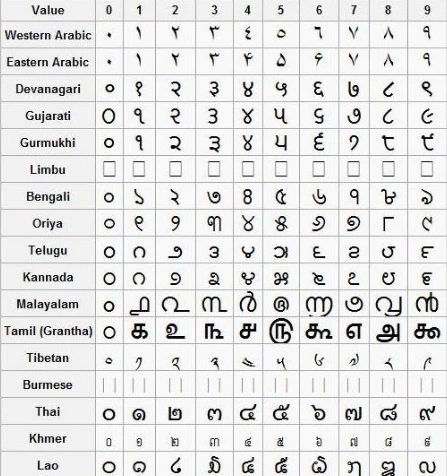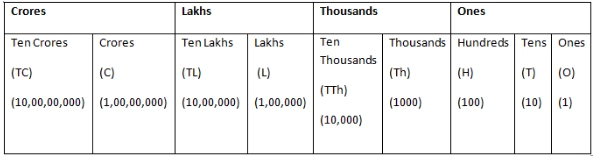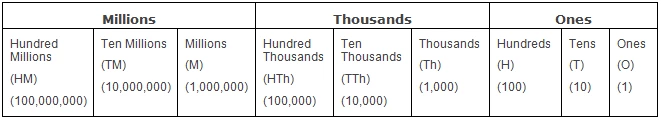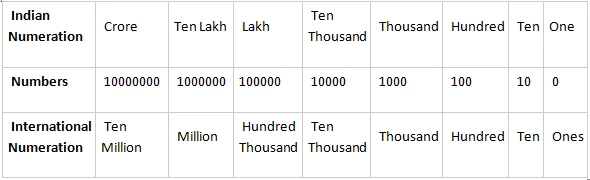Class 6
MATHS
Knowing Our Numbers

# NumerationStep by step solution by experts to help you in doubt clearance & scoring excellent marks in exams.

Updated On: 28-6-2020Apne doubts clear karein ab Whatsapp par bhi. Try it now.Watch 1000+ concepts & tricky questions explained!197.2 K+9.8 K+

Related Videos1527344

45.2 K+

905.7 K+

2:511527343

22.3 K+

447.4 K+

5:291527345

6.3 K+

126.7 K+

2:531721533

1.5 K+

29.9 K+

47:371529524

3.2 K+

65.0 K+

5:221529525

5.5 K+

110.7 K+

3:211529363

98.6 K+

126.4 K+

2:051529362

5.0 K+

100.6 K+

2:494190

66.4 K+

1 M+

4:434192

853.2 K+

1 M+

4:3930918

5.7 K+

114.5 K+

5:0553793499

4.3 K+

86.2 K+

4:09

Very Important Questions

Trending Chapters

Use Of Variables In Geometry And Arithmetics, Ncert Ex-11.2 | WHOLE NUMBERS | Subtraction, NCERT Ex-8.6 | To Find Lcm | UNDERSTANDING ELEMENTARY SHAPES | SYMMETRY | Using Expression Practically. Ex-11.4 | Super Exam Revision of Integers | Part 1 | Whole Numbers|Number Lineproperties Of Whole Numbers | Super Revision- Based on Exam | Part-04

## Numeration

Do you know that before the numbers were invented, some physical objects were used in the counting such as pebbles or sticks? As time progressed, marks on pottery and lines also continued for a long time for counting various things. Then after some time, the numbers came into existence. In the latter time, there was a need to adopt a standard system for counting, that is, a mathematical notation to express numbers with the use of symbols and digits. This process is known as the system of numeration or numeral system. So, across different countries and periods, the numbers developed also differed.  So, for this, we have a large number of numeral systems, e.g., Greek, Roman, Arabic, decimal, binary, etc. In this chapter, we will discuss the Indian and international numeral system. We will look forward to studying numeration where we will discuss numeration system.## Indian Numeral System

In order to understand the Indian numeral system, we will consider a number, say 337. You can notice that the digit 3 has been used twice in this number. You should not that both of them have a different value. So, in this case, we differentiate them by stating their place value. Well, this is defined as the numerical value of a digit on the basis of its position in a number. Thus, in this case, the place value of the leftmost 3 is Hundreds while the one in the center is Tens.

Now, regarding the Indian numeral system, the place values of digits go in the sequence of Ones, Tens, Hundreds, Thousands, Ten Thousand, Lakhs, Ten Lakhs, Crores and so on.

In the number 10,23,45,678 the place values of each digit are:

• 8 – Ones
• 7 – Tens
• 6 – Hundreds
• 5 – Thousands
• 4 – Ten Thousand
• 3 – Lakhs
• 2 – Ten Lakhs
• 0 – Crores
• 1 – Ten Crores

The relationship between them is:

• 1 hundred = 10 tens
• 1 thousand = 10 hundreds = 100 tens
• 1 lakh = 100 thousands = 1000 hundreds
• 1 crore = 100 lakhs = 10,000 thousandsRelated Videos:

## International Numeral System

When it comes to theofit goes in the sequence of Ones, Tens, Hundreds, Thousands, Ten Thousand, Hundred Thousands, Millions, Ten Million and so on, in the international numeral system. The place value if each digit of the number 12,345,678 are:

• 8 – Ones
• 7 – Tens
• 6 – Hundreds
• 5 – Thousands
• 4 – Ten Thousand
• 3 – Hundred Thousands
• 2 – Millions
• 1 – Ten Million

The relations between them are:

• 1 hundred = 10 tens
• 1 thousand = 10 hundreds = 100 tens
• 1 million = 1000 thousand
• 1 billion = 1000 millionsComparison between Indian and International Numeral System

When we compare the two numeral systems we observe that:

• 100 thousands = 1 lakh
• 1 million = 10 lakhs
• 10 millions = 1 crore
• 100 millions= 10 crores## Placement of Comma

In large numbers, commas are placed to help us read and write them easily. When it comes to Indian and international systems, the commas are placed at different positions.

According to the Indian numeral system, the first comma is placed after the hundreds place post which they are placed after every two digits. E.g., 1,23,45,67,890.

Coming to the international numeral system, the first comma is placed after the hundreds place, post which they are placed after every three digits. E.g., 1,234,567,890.

So, we have learnt numeration where we have observed the Indian numeral system as well as International numeral system. We have also clearly understood the comparison between Indian and International Numeral System.

Important Chapters:

By using this site you agree to the use of cookies. Check our Cookie Policy for more details